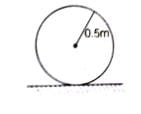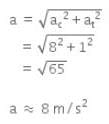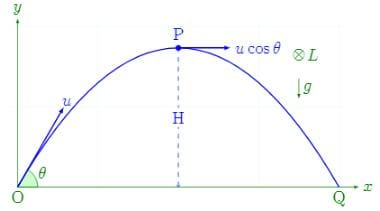NEET  >  Test: Angular Momentum

# Test: Angular Momentum

Test Description

## 10 Questions MCQ Test Physics Class 11 | Test: Angular Momentum

Test: Angular Momentum for NEET 2022 is part of Physics Class 11 preparation. The Test: Angular Momentum questions and answers have been prepared according to the NEET exam syllabus.The Test: Angular Momentum MCQs are made for NEET 2022 Exam. Find important definitions, questions, notes, meanings, examples, exercises, MCQs and online tests for Test: Angular Momentum below.
Solutions of Test: Angular Momentum questions in English are available as part of our Physics Class 11 for NEET & Test: Angular Momentum solutions in Hindi for Physics Class 11 course. Download more important topics, notes, lectures and mock test series for NEET Exam by signing up for free. Attempt Test: Angular Momentum | 10 questions in 10 minutes | Mock test for NEET preparation | Free important questions MCQ to study Physics Class 11 for NEET Exam | Download free PDF with solutions
 1 Crore+ students have signed up on EduRev. Have you?
Test: Angular Momentum - Question 1

### A person standing on a rotating platform with his hands lowered outstretches his arms. The angular momentum of the person

Detailed Solution for Test: Angular Momentum - Question 1

Yes because there is absence of any external force or torque so angular momentum will remain constant
here outstretching the hands means internal forces are working.. so moment of inertia increases in this case and to make angular momentum constant when angular velocity decreases.

Test: Angular Momentum - Question 2

### The mass of an electron is  9 x 10-31 kg. It revolves around the nucleus of an atom in a circular orbit of radius 4.5 Å, with a speed of  8 x 105m/s. The angular momentum of electron is

Detailed Solution for Test: Angular Momentum - Question 2

M=9x10-31Kg,r=4.5x10-10
V=8x105m/s
L=mvr
=9x10-31 x 4.5 x 10-10 x 8 x 10= 3.24 x 10-34 kgm2s-1

Test: Angular Momentum - Question 3

### The earth rotates about an axis passing through its north and south poles with a period of one day. If it is struck by meteorites, then

Detailed Solution for Test: Angular Momentum - Question 3

Because angular momentum remains constant as the moment of inertia is increasing here so the angular velocity will decrease.

Test: Angular Momentum - Question 4

Relation between torque and angular momentum is similar to the relation between

Detailed Solution for Test: Angular Momentum - Question 4

Torque is the rate of change of angular momentum. Force in linear motion corresponds to torque in rotary motion. Thus, just as Newton’s second law can be written as F = dP/dt (F for linear motion), it can be written as T = dL/dt for rotary motion.

Test: Angular Momentum - Question 5

The dimensions of angular momentum are

Detailed Solution for Test: Angular Momentum - Question 5

We know that angular momentum,
L = r x P
Thus its dimension is [r][P] = ML2T-1.

Test: Angular Momentum - Question 6

An earth satellite is moving around the earth in a circular orbit. In such case, what is conserved?

Detailed Solution for Test: Angular Momentum - Question 6

An earth satellite is moving in a circular motion due to change in the direction of velocity. Its angular momentum about centre is conserved as there is no external force acting on the system.

Test: Angular Momentum - Question 7

A uniform circular disc of radius 50 cm at rest is free to turn about an axis which is perpendicular to its plane and passes through its centre. It is subjected  to a torque which produces a constant angular acceleration of 2.0 rad/sec2. It's net acceleration in m/s2 at the end of 2.0 s is a approximately.

Detailed Solution for Test: Angular Momentum - Question 7

A uniform circular disc of radius 50 cm at rest is free to turn about an axis having perpendicular to its plane and passes through its centre. This situation can be shown by the figure given below:Therefore,

Angular acceleration = α = 2 rad/s2

Angular speed, ω = αt = 4 rad/s

Centripetal acceleration, ac = ω2r

42 x 0.5

=16 x 0.5

= 8m/s2

Linear acceleration at the end of 2 s is,

at = αt = 2 x 0.5 = 1 m/s2

Therefore, the net acceleration at the end of 2.0 sec is given byTest: Angular Momentum - Question 8

kgm2s-1 is the unit of

Detailed Solution for Test: Angular Momentum - Question 8

L = Iw
L is the angular momentum, I is the MOI and w is the rotational velocity.
[I] = [ML2T0], [w] = [M0L0T-1]
[L] = [MLT-1]

Test: Angular Momentum - Question 9

Angular momentum is equal to____.(All the symbols used have their usual meanings)

Detailed Solution for Test: Angular Momentum - Question 9

Angular momentum is equal to L = Iω. It is the formula.

Test: Angular Momentum - Question 10

In case of a projectile, the angular momentum is minimum

Detailed Solution for Test: Angular Momentum - Question 10In case of a projectile, the angular momentum is minimum at the starting point.

## Physics Class 11

126 videos|449 docs|210 tests
 Use Code STAYHOME200 and get INR 200 additional OFF Use Coupon Code
Information about Test: Angular Momentum Page
In this test you can find the Exam questions for Test: Angular Momentum solved & explained in the simplest way possible. Besides giving Questions and answers for Test: Angular Momentum, EduRev gives you an ample number of Online tests for practice

## Physics Class 11

126 videos|449 docs|210 tests

### How to Prepare for NEET

Read our guide to prepare for NEET which is created by Toppers & the best Teachers